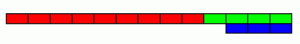Using 3 rods of integer lengths, none longer than 10 units and not using any rod more than once, you can measure all the lengths in whole units from 1 to 10 units. How many ways can you do this?

For example with rods of lengths 3, 4, and 9 the measurements are:

4-3=1; 9-4-3=2; 3; 4; 9-4=5; 9-3=6, 3+4=7; 9+3-4=8; 9 and 9+4-3=10 (as illustrated).Using 3 rods of ANY integer lengths, what is the greatest length N for which you can measure all lengths from 1 to N units inclusive? Can you beat 10 units?

What is the greatest length that can be measured using 4 rods in this way?

Thank you to NRICH for this problem.

Tagged with: